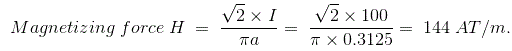# MCQs on Electric Circuits

##### Page 15 of 63. Go to page 1 2 3 4 5 6 7 8 9 10 11 12 13 14 15 16 17 18 19 20 21 22 23 24 25 26 27 28 29 30 31 32 33 34 35 36 37 38 39 40 41 42 43 44 45 46 47 48 49 50 51 52 53 54 55 56 57 58 59 60 61 62 63
01․ What is called a cycle in case of AC operation ?
When ac completes the (+)ve half, is called a cycle.
When ac goes through a complete (+)ve to (-)ve, is called a cycle.
When an alternating quantity completes the (–)ve cycle, is called a cycle.
Option A and C both.

The alternating current changes its magnitude from one instant to another instant continuously with respect to the time interval. Basically the cycle in an alternating current is known as the path of the one half of the positive magnitude and one half of the negative magnitude that starts from the starting point and ends on the ending point.

02․ Four bulbs of 40 W each are connected in series with a battery across them, which of the following statement is true?
The current through each bulb is same.
The voltage across each bulb is not same.
The power dissipation in each bulb is not same.
None of the above.

As the bulb are connected in series, they have same current through them. The voltage across each bulb is same because they have same current and wattage rating. Power dissipation in each bulb is also same. So, only statement A is correct.

03․ Sulphation in a lead acid battery occurs due to
heavy charging.
fast charging.
trickle charging.
incomplete charging.

The sulphation in a lead acid battery occurs due to sudden discharge in a full charged battery. So, it produces more internal resistance and thereby the battery can’t be reached to the fully charged condition. Since, sulphation occurs continuously due to the incomplete charge.

04․ Capacitors for P.f correction are rated in
KW.
KVA.
KV.
KVAR.

The power factor correction is nothing but the reducing the reactive power consumption of an inductive load by generating the apparent power (or) capacitive power. We know that the unit of the apparent power is KVAR. So, the power factor capacitors are also rated in terms of KVAR rating.

05․ Among the options which metal has the highest electrical conductivity?
Aluminium.
Stranded aluminium.
Copper.
Steel.

Copper has the least resistivity (≈ 1.72 × 10-8) value than the other above mentioned metals. So, it has the highest electrical conductivity.

06․ While comparing magnetic field and electric circuits, the point of dissimilarity exists while considering
mmf and emf.
reluctance and resistance.
flux and current flow.
permeance and conductance.

Flow of current in an electric circuit involves continuous expenditure of energy but energy is needed in a magnetic circuit only creating the flux initially but not for maintaining it.

07․ A wire 2.5 m long is bent into a square. An current of 100 A flowing through the wire will produce a magnetizing force at the center of the square, equal to
72 AT / m.
144 AT / m.
196 AT / m.
222 AT / m.

Given data : Wire length L = 2.5 m, Current I = 100 A, Magnetizing force H = ?. In square, length of each side 2a = L / 4 ⇒ a = 2.5 / 8 = 0.3125.08․ Magnetism of a magnet can be destroyed by
heating.
hammering.
by inductive action of another magnet.
by all above methods.

The above all methods could destroy the strength of the magnet.

09․ The mass of the atom is determined by
proton.
electrons.
neutron and proton.
electron and proton.

The mass of the atom is the summation of total mass of neutrons and protons. We do not consider the electrons since the mass of an electron is about 1 / 1800 times the mass of a proton or neutron.

10․ The absolute charge of an electron is
- 1.6 × 10-19 C.
+ 1.6 × 10-19 C.
1.6 × 10-19 C.
none of above.

The electrons have always negative charge. Thus, the absolute charge of an electron is - 1.6 × 10-19 C.

<<<1314151617>>>